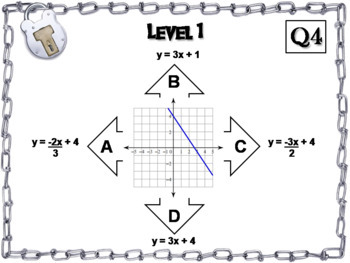Solving Linear Equations Game: Algebra Escape Room Math ActivitySubject
Resource Type
Product Rating
File Type

PDF (Acrobat) Document File

1 MB|15 pages
Share
Product Description
This breakout escape room is a fun way for students to test their skills with linear equations.

Contents:

♦ Teacher Instructions and FAQ
♦ 3 Levels to decode: Maze Decoder, Tarsia Puzzle, and Message Decoder
♦ Level 1: Students find the slope-intercept form from a graph
♦ Level 2: Students find the slope-intercept form from standard form
♦ Level 3: Students find the standard form from coordinate points and the slope
♦ Student Recording Sheet and Teacher Answer Key
♦ Link to an optional, but recommended, digital breakout room

Check out the preview for more details!

Important: If you enjoyed this product, check out my other Math Escape Rooms:
Note: Each topic utilizes the same types of puzzles

Algebra: Get all 56 (50% OFF) in the Bundle!
Absolute Value Equations
Absolute Value Inequalities
Arithmetic and Geometric Sequences
Combinations and Permutations
Combining Like Terms
Complex Numbers
Compound Interest
Dimensional Analysis
Equations of Lines
Evaluating Expressions
Exponential Growth and Decay
Exponents - Dividing
Exponents - Multiplying
Exponents - Multiplying and Dividing
Function Operations
Greatest Common Factor of Monomials
Greatest Common Factor of Polynomials
Linear Equations
Literal Equations
Logarithms
Literal Equations
Monomials: Dividing
Monomials: Multiplying
Monomials: Multiplying & Dividing
Multi-Step Equations
Multi-Step Inequalities
One and Two Step Equations
One and Two Step Inequalities
Parallel and Perpendicular Lines
Polynomials: Factoring
Polynomials - Multiplying and Dividing
Polynomials - Operations with
Proportions
Rational Expressions: Multiplying and Dividing
Rational Expressions: Multiplying
Rational Expressions: Operations with
Rational Expressions: Simplifying
Scientific Notation: Multiplying and Dividing
Scientific Notation: Operations with
Simple and Compound Interest
Simplifying Expressions
Slope
Systems of Equations by Elimination
Systems of Equations by Substitution
Writing Equations from Word Problems

Geometry: Get all 13 (40% OFF) in the Bundle!
Angles/ Slopes of Parallel and Perpendicular Lines
Area and Circumference of a Circle
Area and Perimeter (2D Shapes)
Area of Composite Figures
Congruent Triangles
Equations of Lines
Midpoint and Distance Formula
Missing Angles
Missing Angles of Triangles
Pythagorean Theorem
Special Right Triangles
Surface Area and Volume

Grades 6-8: Get all 11 (55% OFF) in the Bundle!
Equivalent Fractions
Integers: Multiplying and Dividing
Mixed Numbers and Improper Fractions
Mixed Numbers: Multiplying and Dividing
Negative Exponents
Percent Change Word Problems
Proportions Word Problems
Rational Numbers: Multiplying and Dividing
Ratios
Reducing Fractions
Repeating Decimals to Fractions
Simple Interest
Percents, Decimals, Fractions
Unit Rate Word Problems

Grades 3-5: Get all 34 (55% OFF) in the Bundle!
Capacity
Balancing Equations
Decimal Place Value
Decimals: Multiplying and Dividing
Elapsed Time
Expanded Form
Exponents
Fact Families
Factors and Multiples
Fractions: Dividing by Whole Numbers
Fractions: Multiplying and Dividing
Fractions: Multiplying by Whole Numbers
Fractions Word Problems
Greatest Common Factor
Least Common Multiple
Mean, Median, Mode, Range
Metric Measurement
Multi-Digit Multiplication
Multiplication Word Problems
Multi-Step Word Problems
Number Patterns
Order of Operations
Percents
Place Value
Prime Factorization
Probability
Roman Numerals
Simplifying Fractions
Two-Digit Multiplication
Two-Step Word Problems

Grades 1-3: Get all 12 (35% OFF) in the Bundle!
Counting Coins
Fact Families
Long Division
Multiplication Word Problems
Skip Counting
Subtraction: 2 Digit
Telling Time
Word Problems: Addition and Subtraction With Regrouping
Word Problems: Addition and Subtraction Without Regrouping
Total Pages
15 pages